## Markov Chain Modeling

### Discrete-Time Markov Chain Object Framework Overview

The `dtmc` object framework provides basic tools for modeling and analyzing discrete-time Markov chains. The object supports chains with a finite number of states that evolve in discrete time with a time-homogeneous transition structure.

`dtmc` identifies each Markov chain with a `NumStates`-by-`NumStates` transition matrix P, independent of initial state x0 or initial distribution of states π0. You can specify P as either a right-stochastic matrix or a matrix of empirical counts.

• As a right-stochastic matrix:

• Pij is the nonnegative probability of a transition from state i to state j.

• Each row of P sums to 1.

• ${\pi }_{t+1}={\pi }_{t}P$ describes the evolution of the state distribution from time t to time t + 1.

The state distribution at time t, πt is a row vector of length `NumStates`.

• As a matrix of empirical counts, Pij is the observed number of times state i transitions to state j. The `dtmc` object normalizes the rows of P so that it is a right-stochastic matrix.

The `mcmix` function is an alternate Markov chain object creator; it generates a chain with a specified zero pattern and random transition probabilities. `mcmix` is well suited for creating chains with different mixing times for testing purposes.

To visualize the directed graph, or digraph, associated with a chain, use the `graphplot` object function. `graphplot` is similar to the `plot` object function of a MATLAB® `digraph` object, but it includes additional functionality for analyzing Markov chain structure. Parameter settings highlight communicating classes (that is, strongly connected components of the digraph) and specific characteristics affecting convergence, such as recurrence, transience, and periodicity. You can highlight transition probabilities in P by coloring the graph edges using heatmap intensities.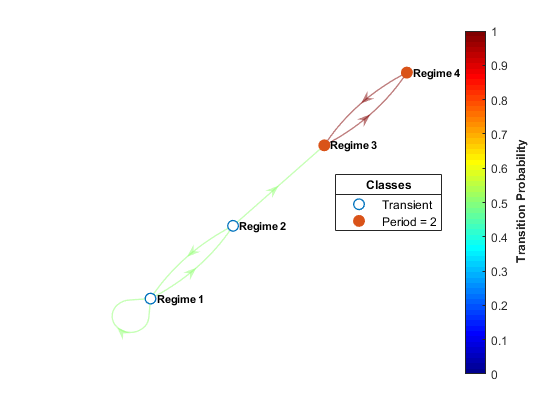To visualize large-scale structure in the chain, `graphplot` can condense communicating classes to representative nodes. This option is based on the `condensation` object function of a `digraph` object.

The `classify` object function is a numerical analog of class highlighting in the graph. `classify` returns characteristics of the communicating classes that determine limiting behavior. State classification combines graph-theoretic algorithms, such as the `bfsearch` (breadth-first search) object function of a MATLAB `graph` object, but with more direct matrix computations specific to Markov chain theory. The `subchain` method allows you to extract specific communicating classes from the chain for further analysis.

The `isreducible` and `isergodic` object functions give concise summaries of chain structure. Together, they provide necessary and sufficient conditions for the existence of a unique limiting distribution ${\pi }^{\ast }$, where ${\pi }^{\ast }={\pi }^{\ast }P$ and ${\pi }_{0}\to {\pi }^{\ast }$ for every initial distribution π0. The `asymptotics` object function computes ${\pi }^{\ast }$, if it exists, and estimates the mixing time using eigenvalue analysis. The `eigplot` object function plots the eigenvalues of P. This figure shows an example of an eigenvalue plot returned by `eigplot`.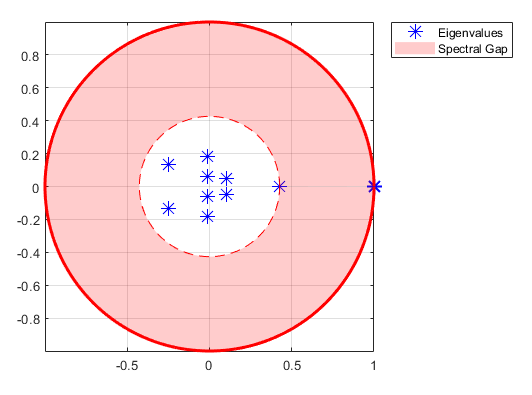One obstacle to convergence is periodicity. The `lazy` object function eliminates periodicity by adjusting state inertia (that is, by weighting the diagonal elements of P) to produce specified amounts of “laziness” in the chain. Limiting distributions are unaffected by these transformations.

The `simulate` and `redistribute` object functions provide realizations of the process as it evolves from a specified initial state or distribution. The `simplot` and `distplot` object functions provide various visualizations. This figure is an example of a distribution plot showing the evolution of the state distribution starting from a uniform initial state distribution.### Markov Chain Analysis Workflow

You can start building a Markov chain model object in two ways:

• Identify pertinent discrete states in a process, and then estimate transition probabilities among them. In the simplest case, theory suggests chain structure and the transition matrix P. In this situation, you are interested primarily in how the theory plays out in practice—something that is not always obvious from theory. Once you know P, create a Markov chain object by passing P to `dtmc`, which implements a theoretical chain.

• If you have less specific information on a process, then you must experiment with various numbers of states and feasible transition patterns to reproduce empirical results. The `mcmix` function provides insight into the skeletal structure of a chain, which can capture essential features in the data. Through an iterative process, you can adjust the randomly generated transition matrix P to suit modeling goals.

For an econometric model builder, the most important consequence of the choice of P is the asymptotic behavior of the chain. To understand this behavior, identify and separate the transient states (those states whose return-time probabilities go to zero asymptotically) from the recurrent states (those states whose return-time probabilities go to one asymptotically). Transience and recurrence are properties shared by all states in a communicating class. To determine visually whether states are transient or recurrent, pass the Markov chain object to the `graphplot` object function and specify `'ColorNodes',true`. Alternatively, the outputs of the `classify` object function provide numerical tools for evaluation. This figure is an example of a digraph with classified nodes.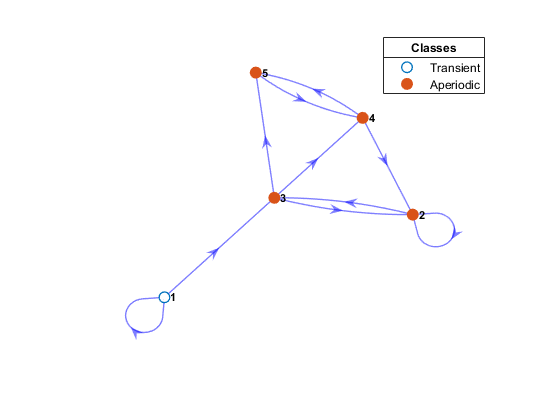The condensed view of the digraph simplifies this evaluation by consolidating each communicating class into a “supernode.” In the condensed graph, you can easily recognize transience and recurrence by the out degree of the supernode (an out degree greater than 0 implies transience). Irreducible chains consist of a single, necessarily recurrent, communicating class. Unichains consist of a single recurrent class and any number of satellite transient classes. Unichains maintain the desirable limiting behavior of an irreducible chain. Consideration of the condensed graph is often a precursor to trimming a chain of irrelevant transient states. The `subchain` function trims chains of transient classes. This figure is the condensed view of the digraph in the previous figure.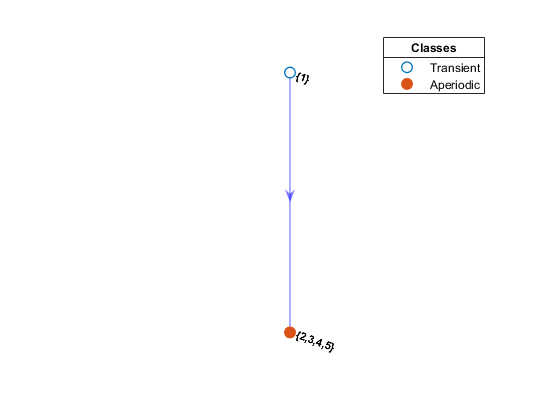The two principle obstacles to uniform limiting behavior are:

• Reducibility, the existence of more than one communicating class

• Periodicity, the tendency to cycle among subclasses within a single class

A combination of the `graphplot` and `classify` object functions can identify these issues. If a chain is reducible and not a unichain, it is common to split the analysis among the independent recurrent classes or reformulate the chain altogether. If a chain is periodic (that is, it contains a periodic recurrent class), but the overall structure captures the essential details of an application, the `lazy` object function provides a remedy. Lazy chains perturb the diagonal elements of P to eliminate periodicity, leaving asymptotics unaffected.

The `isreducible` and `isergodic` object functions summarize state classification. Every chain has a stationary distribution ${\pi }^{\ast }$, where ${\pi }^{\ast }={\pi }^{\ast }P$, as a result of P being stochastic and having an eigenvalue of one. If the chain is irreducible, the stationary distribution is unique. However, irreducibility, while sufficient, is not a necessary condition for uniqueness. Unichains also lead to a unique stationary distribution having zero probability mass in the transient states. In this regard, state classification analysis is essential because `isreducible` returns `true` only if the chain as a whole consists of a single communicating class. `isreducible` returns `false` for arbitrary unichains, in which case you must decide whether transient classes are a relevant part of the model.

Ergodicity, or primitivity, is the combination of irreducibility and aperiodicity. An ergodic chain has a unique limiting distribution, that is, π0 converges to ${\pi }^{\ast }$ for every initial distribution π0. You can determine whether the chain, as a whole, is ergodic by using `isergodic`. The function identifies ergodic unichains by evaluating the sole recurrent class. A chain is periodic if it is irreducible and not ergodic, that is, if `~tfirreduc` + `~tfergo` = `false`, where `tfirreduc` and `tfergo` are returned by `isreducible` and `isergodic`, respectively.

Once you have confirmed that a chain is ergodic, you can determine the unique limiting distribution by using the `asymptotics` object function. `asymptotics` returns the limiting distribution ${\pi }^{\ast }$ and an estimate of the mixing time, which is a time constant for the decay of transient behavior. The Perron-Frobenius theorem for irreducible nonnegative matrices (see ) is useful for interpreting these results. Any stochastic matrix has a spectral radius of one. Periodic matrices, of period k, have k eigenvalues distributed uniformly around the unit circle at the k roots of unity. The magnitude of the largest eigenvalue inside the unit circle determines the decay rate of transient states. The `eigplot` object function provides quick visualization of this information. This figure is an eigenvalue plot of a Markov chain with a period of three.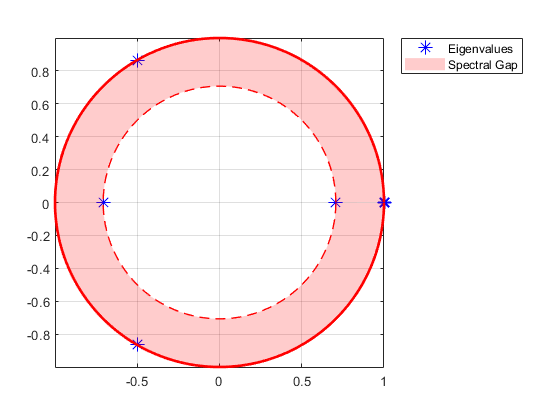Regardless of the asymptotic properties of the chain, you can study its mixing rate by applying finite-step analysis. The `hitprob` and `hittime` functions return hitting probabilities and expected first hitting times for a subset of target states, beginning from each state in the chain. Both functions optionally plot a digraph with node colors specifying the hitting probabilities or times. This figure shows an example of a digraph with node colors specifying the expected first hitting times. The digraph also indicates whether the beginning states are remote for the target.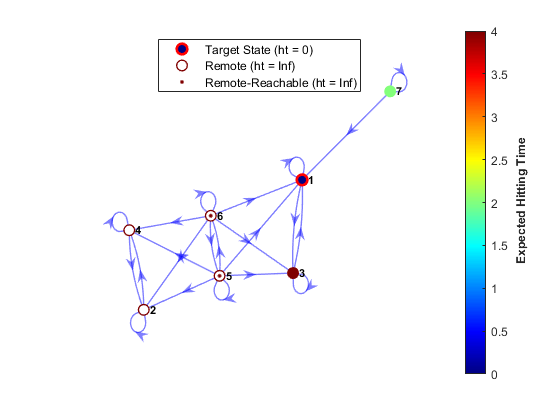Simulation and redistribution allow you to generate statistical information on the chain that is difficult to derive directly from the theory. The `simulate` and `simplot` object functions, and the `redistribute` and `distplot` object functions, provide computational and graphical tools for such analyses. `simulate`, for example, generates independent random walks through the chain. As with `simulate` and object functions elsewhere in Econometrics Toolbox™, ensemble averages of dependent statistics play an important role in forecasting. The corresponding `simplot` object function offers several approaches to visualization. This figure displays the proportion of states visited after 100 random walks of length 10 steps through the periodic Markov chain in the previous figure.Horn, R., and C. R. Johnson. Matrix Analysis. Cambridge, UK: Cambridge University Press, 1985.